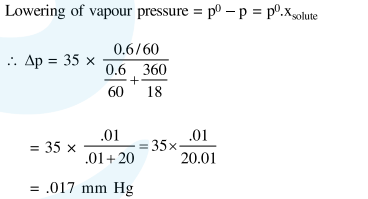Deepak Scored 45->99%ile with Bounce Back Crack Course. You can do it too!

# At room temperature, a dilute soluton of urea is prepared by dissolving

Question:

At room temperature, a dilute soluton of urea is prepared by dissolving $0.60 \mathrm{~g}$ of urea in $360 \mathrm{~g}$ of water. If the vapour pressure of pure water at this temperature is $35 \mathrm{mmHg}$, lowering of vapour pressure will be (molar mass of urea $=60 \mathrm{~g} \mathrm{~mol}^{-1}$ ):-

1. $0.027 \mathrm{mmHg}$

2. $0.028 \mathrm{mmHg}$

3. $0.017 \mathrm{mmHg}$

4. $0.031 \mathrm{mmHg}$

Correct Option: , 3

Solution: